Thermodynamics and Propulsion

# 2.1 First Law of Thermodynamics

[VW, S & B: 2.6]

Observation leads to the following two assertions:

1. There exists for every system a property called energy,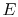. The system energy can be considered as a sum of internal energy, kinetic energy, potential energy, and chemical energy.
1. Like the Zeroth Law, which defined a useful property, temperature,'' the First Law defines a useful property called energy.''
2. The two new terms (compared to what you have seen in physics and dynamics, for example) are the internal energy and the chemical energy. For most situations in this class, we will neglect the chemical energy. We will generally not, however, neglect the internal energy,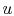. It arises from the random or disorganized motion of molecules in the system, as shown in Figure 2.1. Since this molecular motion is primarily a function of temperature, the internal energy is sometimes called thermal energy.''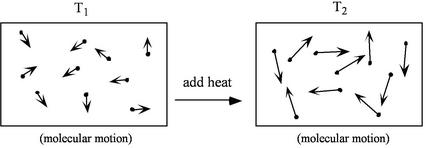The internal energy,, is a function of the state of the system. Thus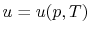, or, or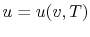. Recall that for pure substances the entire state of the system is specified if any two properties are specified. (We will discuss the equations that relate the internal energy to these other variables as the course progresses.)

2. The change in energy of a system is equal to the difference between the heat added to the system and the work done by the system,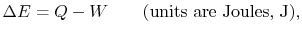(2..1)

whereis the energy of the system,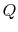is the heat input to the system, and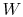is the work done by the system.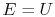(thermal energy) +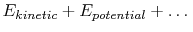1. Like the Zeroth Law, the First Law describes the behavior of the new property [VW, S& B: Chapter 5].
2. The equation can also be written on a per unit mass basis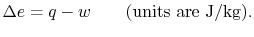3. In many situations the potential energy, kinetic energy, and chemical energy of the system are constant or not important. Then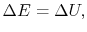and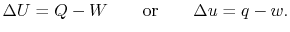4. Note thatandare not functions of state, but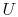, which arises from molecular motion (see above), depends only on the state of the system;does not depend on how the system got to that state. We therefore have the striking result that:Sometimes this difference is emphasized by writing the First Law in differential form,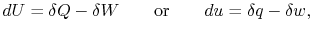(2..2)

where the symbol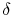'' is used to denote that these are not exact differentials but rather are dependent on path.
5. Note that the signs are important:
•is defined to be positive if it is transferred to the system; thus the numerical value we substitute forwill be positive if heat is transferred to the system from the surroundings, and negative if heat is transferred from the system to the surroundings. [VW, S & B: 4.7-4.8]
•is defined to be positive if it is done by the system (see Section 1.3); thus the numerical value we substitute forwill be positive if the system is doing work, and negative if work is being done on the system. [VW, S& B: 4.1-4.4]
6. For quasi-static processes we can substitute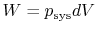,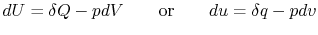To give an example of where the first law is applied, consider the device shown in Figure 2.2. We heat a gas, it expands against a weight, some force (pressure times area) is applied over a distance, and work is done. The change in energy of the system supplies the connection between the heat added and work done. We will spend most of the course dealing with various applications of the first law -- in one form or another.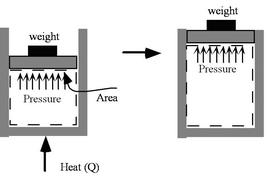The form of the first law we have given here is sometimes called the control mass'' form, because it is well suited to dealing with systems of a fixed mass. We will see in Section 2.5 that this form can be written for a control volume with mass flow in and mass flow out (like a jet engine for example). We will call this the control volume'' form of the first law [VW, S & B: 5.8-5.12].

Muddy Points

What are the conventions for work and heat in the first law? (MP 2.1)

When does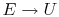? (MP 2.2)

Douglas Quattrochi 2006-08-06